Published: 01 December 2017

# Vibroacoustic handling and levitation of microparticles in air

Vytautas Ostasevicius1
Vytautas Jurenas2
Rimvydas Gaidys3
1, 2, 4Institute of Mechatronics, Kaunas University of Technology, Kaunas, LT51424, Lithuania
3Department of Mathematical Modeling, Kaunas University of Technology, Kaunas, LT51424, Lithuania
Corresponding Author:
Vytautas Ostasevicius
Views 281

#### Abstract

The levitation and controlled movement of substances in the air has many potential applications, from materials handling to biochemistry and pharmacy. In this work, the handling of microparticles by sound field in vibrating cylinder was investigated by simulation and experimental measurement. A standing wave field created between the piezotransducer and the reflector created the conditions for levitating microparticles, which were concentrated at nodes of the vibrating cylinder surface. The acoustic wave and the cylinder walls were excited by the same disk-shaped piezotransducer fixed to the bottom of the cylinder

## 1. Introduction

The noncontact handling of small particles around the circular trajectory was investigated in . A circular aluminum plate with a piezoelectric ring was used as a vibrating plate. According to FEA calculations, the piezoelectric ring electrodes were divided into 24 units to generate flexural vibration mode with one nodal circle and four nodal lines at 47.8 kHz resonance frequency. The vibrator is fitted with a circular plate, the size of which is the same as the vibrating plate. It was used as a reflector to generate an acoustic standing wave in the air between two plates. The acoustic field between the vibrating plate and the reflector was calculated by the FEA, and the distribution of the force of acoustic radiation on the microparticle was calculated to predict the position of the trapped particle. Using a prototype of a vibrating plate, polystyrene particles may be trapped at regular intervals along the horizontal nodal line of standing wave. By switching the driving conditions of the divided electrodes in the direction of the circle, the vibration plate nodal lines can be rotated, and trapped particles can be manipulated with a circular trajectory in air. In  an acoustophoretic concept for material contactless transport and handling in air is presented. Spatiotemporal modulation of the levitation acoustic field allows continuous processing and transport of several objects from close to spherical to wire type, but not limited to acoustic wavelength. The independence of the work principle from the properties of special materials (magnetic, optical or electrical) is illustrated using a wide application experiment palette. Noncontact manipulation techniques are useful for micromachinery technology, biotechnology and new material processing. In the paper  advanced manipulation technique for the transport of small objects in the air is described. The standing wave has been created by two sound beams that cross each other, created by bolted Langevin transducers. The expanded polystyrene particles were trapped in the sound pressure nodes in a standing wave field. The trapped particle position was shifted by changing the phase difference between the two sound beams. When the trapped particle is transported, it spatially oscillates in a direction perpendicular to the transportation of particles. Calculating the numerical acoustic field, it was shown that this results in the reflection of the ultrasound wave on each transducer surface. Ultrasonic particle manipulation tools have many promising applications in the field of life sciences, developing the capabilities of current manipulation technologies. In the paper , ultrasonic manipulation of particles and cells by microfluidic channel with piezoelectric array is demonstrated. An array integrated into a flat, multilayer resonator structure directs the particles towards the pressure nodal plane along the center line of the channel, and then to the maximum acoustic velocity above the subset of the active elements. The sound can levitate objects of various sizes and materials through air, water and tissue. It allows to manipulate cells, liquids, compounds or living things without touching or without contamination. However, acoustic levitation required the targets to be enclosed with acoustic elements or had limited maneuverability. The phases used to drive an ultrasonic phased array and show that acoustic levitation can be used to translate, rotate, and manipulate particles using even one-sided emitter are optimized in . In addition, a holographic structure of acoustic elements that allows rapid trapping and bridges between optical and acoustic traps is presented. Single-beam levitation can manipulate particles inside our body for targeted drug delivery or acoustically controlled micro-machines that do not interfere with the imaging of magnetic resonance.

From the literature review, we see that ultrasound vibrations are commonly used for the transport of microparticles, and they are levitated using ultrasound waves. Most of the research works are devoted only to the transport of microparticles, or to their levitation. We propose the use of non-ultrasonic acoustic waves propagating in a cylinder, which, in its own form, is excited from the same piezoelectric transducer for acoustic waves excitation.

## 2. Methods

In acoustic levitation, there is a steady wave between the emitting surface and the reflector. The radiation pressure, the non-linear properties of the acoustic field, leads to the levitation potential-amount of acoustic potential and gravitational potential. It differs nonmonotonically between the radiating surface and the reflector. If it's strong enough to overcome the gravitational force, a small amount of material can be levitated and trapped. The acoustic potential node may correspond to the acoustic pressure node or antinode depending on the density and compression of the levitated material and the surrounding medium. The concept of acoustic levitation and handling is realized using vibrating cylinder, the vibrations of which are excited by a disk-shaped piezo actuator placed at the bottom, which at the same time causes an acoustic wave that reflects from the reflector displayed at the other end of the cylinder (Fig. 1). The resulting standing wave acoustic leads to the levitation of microparticles concentrated on the nodal points of the vibrating cylinder surface. In this way, it is sufficient to excite the sonic, rather than ultrasonic frequency signal, that the microparticles could be levitated from the cylinder surface.

Fig. 1Experimental setup: 1 – cylinder resonant waveguide, 2 – disc-shape piezoelectric bimorph, 3 –microparticles, 4 – signal generator (Agilent 33220A), 5 – linear amplifier EPA-104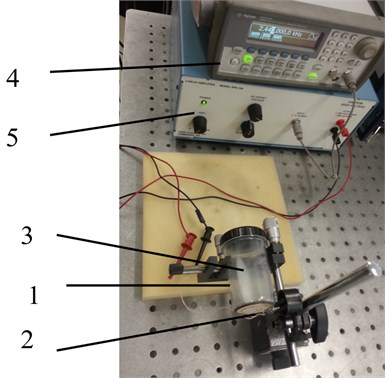## 3. Results

During the experiment, the movement of microparticles was observed, which proves that the formation of ribs is caused by air vortex motion caused by acoustic stationary waves. The microparticles are aggregated in ribs at the pressure nodes of the acoustic wave in the waveguide. This results in circular motion of the air on both sides of the microparticles ribs. The vortex formation causes the regular levitating structures of microparticles (Fig. 2).

Fig. 2Photos of levitating microparticles on the vibrating cylinder wall nodal sections at different excitation frequencies: a) 2.44 kHz, b) 14.2 kHz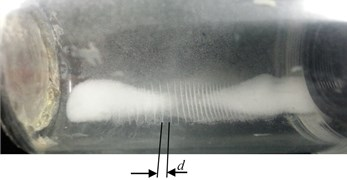a)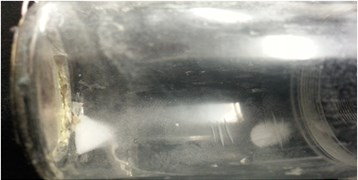b)

Experimental results show that at frequencies over 2 kHz the distance $d$ between microparticles ribs is stable and equal 3-5 mm. The vortex intensity is proportional to flow velocity of the air. Thus, the highest ribs are formed at the sound pressure zones where it’s velocity is maximum. At the sound velocity nodes there are no ribs because the air doesn’t move there. The distance between the ribs depends on frequency because of simple energy and angular momentum conversation. With increasing frequency, the air velocity also increases, this means that the vortices of the air spin faster. The angular momentum conservation can be expressed, as:

1
${\omega }_{0}{r}_{0}^{2}=\omega {r}^{2},$

where ${\omega }_{0}$– initial angular velocity; ${r}_{0}$ – initial vortex radius; $\omega$ – angular velocity at higher frequency; $r$ – vortex radius at higher frequency. If vortex angular velocity $\omega$ is proportional to acoustic wave frequency $f$, Eq. (1) could be expressed as:

2
$r-{r}_{0}{\left(\frac{{f}_{0}}{f}\right)}^{0,5}.$

Comparison with the experimental data yields function $r=a{f}^{b}$ graphically presented in Fig. 3,

where $a=$ 740 and $b=$ –0,5 are experimentally determined parameters, and $r\ll \lambda$, where $\lambda$ acoustic wave length.

The acoustic levitation and handling concept is based on the wave equation theory, the solution as a sum of forward and backward propagating acoustic waves is expressed as following :

3
$p\left(x\right)=\frac{{P}_{m}}{2\mathrm{s}\mathrm{i}\mathrm{n}\left(\frac{n\pi x}{{\lambda }_{n}}-2\pi ft\right)}+\frac{{P}_{m}}{2\mathrm{s}\mathrm{i}\mathrm{n}\left(\frac{n\pi x}{{\lambda }_{n}}+2\pi ft\right)},$

where $p\left(x\right)$ is the sound pressure; ${P}_{m}=$ 2$A$ is the peak sound pressure amplitude in waveguide; $A$ is the vibration amplitude of piezoelectric trasducer; ${\lambda }_{n}$ is a waveguide length; $f$ – frequency of acoustic field; $n$ – harmonic number; $x$ is the space variable; $t$ is the time variable. Eq. (3) indicates that the superposition of the forward and reflected sinusoidal waves is the localized wavepacket represented as the sound pressure evolution in time and space. It is obvious that the sum of two sine functions belongs to new kind of solution rather than simple sine function. Because the size of microparticles is much smaller than the wavelength of generated acoustic standing wave (30 mm-300 mm), the microparticles exposed in the acoustic field are subjected to the action of acoustic radiation force.

Fig. 3The dependence of the distance between the microparticles ribs and the sound frequency, according calculation data and experimental results fit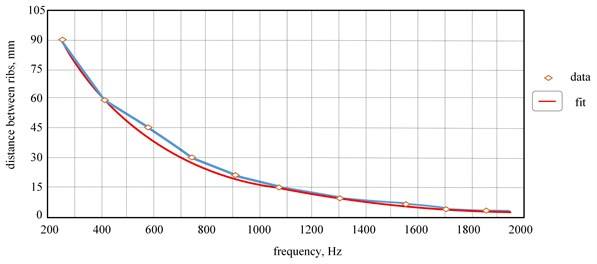Fig. 4Acoustic pressure field in cylinder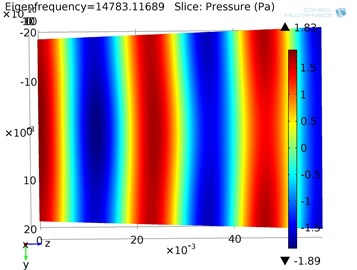Fig. 5Acoustic pressure dependence along the cylinder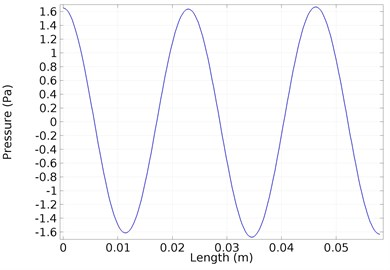Fig. 6Sound pressure level in the cylinder section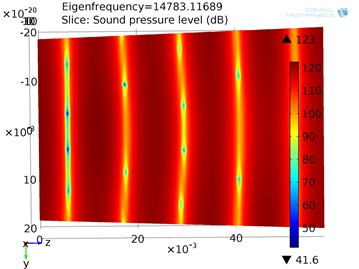Fig. 7Sound pressure level distribution on the cylinder surface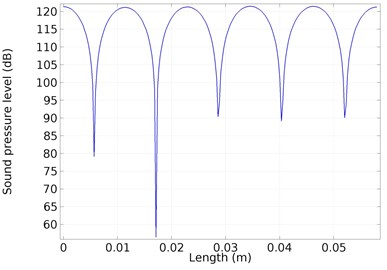The organic glass cylinder with a lid, the bottom of which is a piezotransducer is filled with air. Cylinder is a deformable body in which piezotransducers vibrations generate mechanical vibrations and acoustic pressure fields. This physical phenomenon describes the physics of the deformable body and the acoustic field, why it is necessary to examine the connected physical system and to simulate the corresponding task. This task joining the deformable body and acoustic field physics was been realized in the COMSOL multiphysics environment using a numerical finite element method. The experimental study found that the system’s resonant frequency is 14200 Hz. After modal analysis, from the results obtained (Fig. 4) it can be seen that the acoustic field has three clearly defined zones of high and low pressure, which repeat successively.

The dependence of this pressure on the cylinder is given in Fig. 5, which shows that we have two and a half waves.

The distribution of the sound pressure level is presented in Figs. 6, 7 and 8, respectively.

We see that the lowest level of sound pressure level matches the value of the zero acoustic field.

Fig. 8Sound pressure level field on the cylinder surface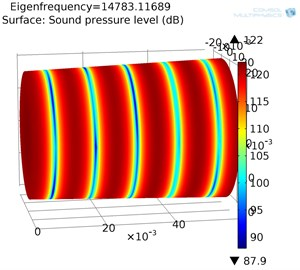## 4. Conclusions

The paper shows the possibility of controlling microparticles by piezoelectric device, causing their levitation phenomena under the influence of acoustic standing waves and cylinder modes of vibration. The proposed method allows that micro- and nanoparticle flows in air be controlled by unique piezoelectric actuator mounted at the tip of a cylindrical cylinder. The concept of the method is demonstrated in a short cylinder experimentally and numerically.

07 November 2017
Accepted
15 November 2017
Published
01 December 2017
SUBJECTS
Acoustics, noise control and engineering applications
Keywords
finite element analysis
experimental setup
acoustic standing wave
vibrating cylinder
Acknowledgements

This work was funded by a Grant (No. SEN-10/15) from the Research Council of Lithuania. Project acronym: “CaSpine”.